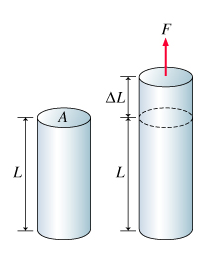# Problem: Learning Goal: To understand the meaning of Young's modulus and to perform some real-life calculations related to the stretching of steel.Hooke's law states that for springs and other "elastic" objectsF = K•Δxwhere F is the magnitude of the stretching force, Δx is the corresponding elongation of the spring from equilibrium, and k is a constant that depends on the geometry and the material of the spring. If the deformations are small enough, most materials, in fact, behave like springs: Their deformation is directly proportional to the external force. Therefore, it may be useful to operate with an expression that is similar to Hooke's law but describes the properties of various materials, as opposed to objects such as springs.Consider, for instance, a bar of initial length L and cross-sectional area A stressed by a force of magnitude F. As a result, the bar stretches by ΔL.Let us define two new terms:Tensile stress is the ratio of the stretching force to the cross-sectional area:Stress = F/ATensile strain is the ratio of the elongation of the rod to the initial length of the bar:Strain = ΔL/LIt turns out that the ratio of the tensile stress to the tensile strain is a constant as long as the tensile stress is not too large. That constant, which is an inherent property of a material, is called Young's modulus and is given by:Y = (F/A)/(ΔL/L)(a) What is the SI unit of Young's modulus?(b) Consider a metal bar of initial length L and cross-sectional area A. The young's modulus of the material of the bar is Y. Find the "spring constant" k of such a bar for low values of tensile strain.

###### FREE Expert Solution

(a)

$\begin{array}{rcl}\mathbf{Y}& \mathbf{=}& \frac{\mathbf{F}\mathbf{/}\mathbf{A}}{\mathbf{∆}\mathbf{L}\mathbf{/}\mathbf{L}}\\ & \mathbf{=}& \frac{\mathbf{N}\mathbf{/}{\mathbf{m}}^{\mathbf{2}}}{\overline{)\mathbf{m}}\mathbf{/}\overline{)\mathbf{m}}}\end{array}$

94% (332 ratings)###### Problem DetailsLearning Goal: To understand the meaning of Young's modulus and to perform some real-life calculations related to the stretching of steel.

Hooke's law states that for springs and other "elastic" objects

F = K•Δx

where F is the magnitude of the stretching force, Δx is the corresponding elongation of the spring from equilibrium, and k is a constant that depends on the geometry and the material of the spring. If the deformations are small enough, most materials, in fact, behave like springs: Their deformation is directly proportional to the external force. Therefore, it may be useful to operate with an expression that is similar to Hooke's law but describes the properties of various materials, as opposed to objects such as springs.

Consider, for instance, a bar of initial length L and cross-sectional area A stressed by a force of magnitude F. As a result, the bar stretches by ΔL.

Let us define two new terms:

Tensile stress is the ratio of the stretching force to the cross-sectional area:
Stress = F/A

Tensile strain is the ratio of the elongation of the rod to the initial length of the bar:
Strain = ΔL/L

It turns out that the ratio of the tensile stress to the tensile strain is a constant as long as the tensile stress is not too large. That constant, which is an inherent property of a material, is called Young's modulus and is given by:

Y = (F/A)/(ΔL/L)

(a) What is the SI unit of Young's modulus?

(b) Consider a metal bar of initial length L and cross-sectional area A. The young's modulus of the material of the bar is Y. Find the "spring constant" k of such a bar for low values of tensile strain.